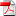# A Non-Intrusive Stratified Resampler for Regression Monte Carlo: Application to Solving Non-Linear Equations

27
Sep

EMMANUEL GOBET, GANG LIU, AND JORGE P. ZUBELLI
Abstract. Our goal is to solve certain dynamic programming equations associated to a given Markov chain X, using a regression-based Monte Carlo algorithm. More speci cally, we assume that the model for X is not known in full detail and only a root sample X1; : : : ;XM of such process is available. By a strati cation of the space and a suitable choice of a probability measure , we design a new resampling scheme that allows to compute local regressions (on basis functions) in each stratum. The combination of the strati cation and the resampling allows to compute the solution to the dynamic programming equation (possibly in large dimensions) using only a relatively small set of root paths. To assess the accuracy of the algorithm, we establish non-asymptotic error estimates in L2(). Our numerical experiments illustrate the good performance, even with M = 20 40 root paths.
Key words. discrete Dynamic Programming Equations, empirical regression scheme, resampling methods, small-size sample

•rr-fime-16-10